• +91 9971497814
• info@interviewmaterial.com

# Chapter 3 Trigonometric Functions Ex-3.3 Interview Questions Answers

### Related Subjects

Question 1 : Prove that: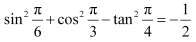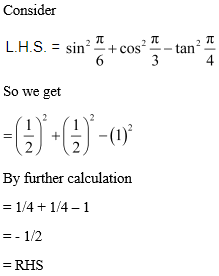Question 2 : Prove that: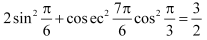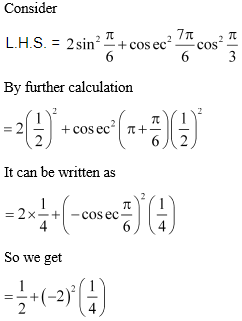Here

= 1/2 + 4/4

= 1/2 + 1

= 3/2

= RHS

Question 3 : Prove that: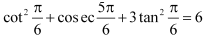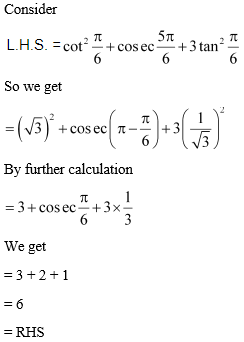Question 4 : Prove that: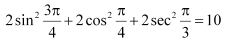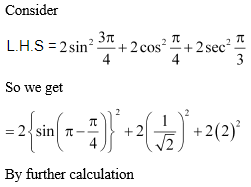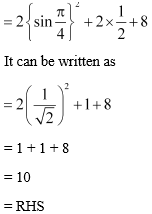Question 5 :
Find the value of:
(i) sin 75o
(ii) tan 15o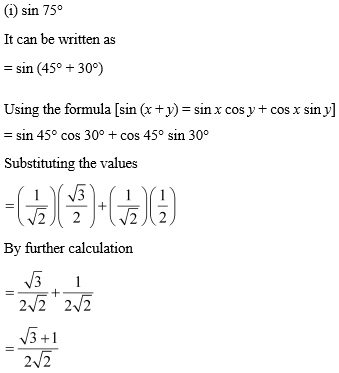(ii) tan 15°

It can be written as

= tan (45° – 30°)

Using formula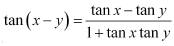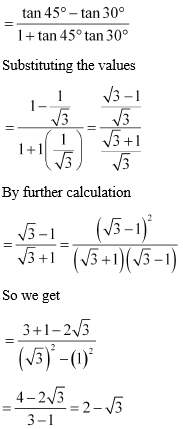Question 6 : Prove that: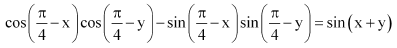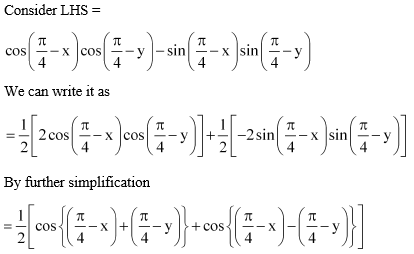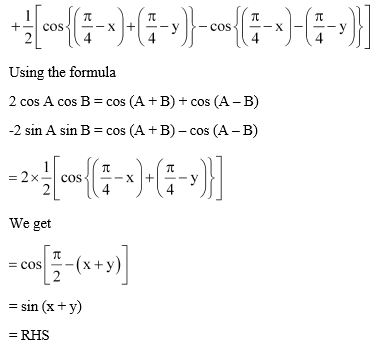Question 7 : Prove that: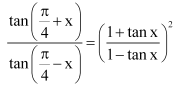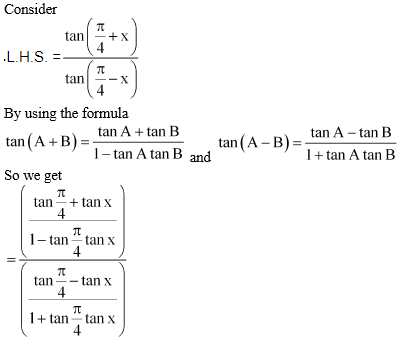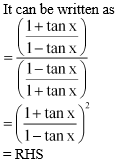Question 8 : Prove that: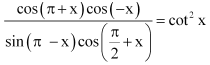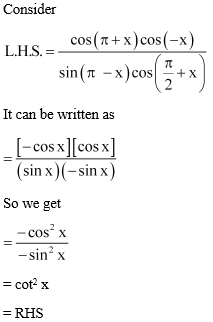Question 9 : Prove that: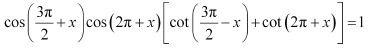Consider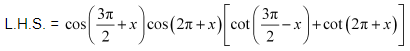It can be written as

= sin x cos x (tan x + cot x)

So we get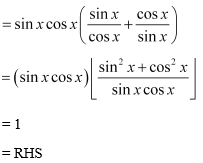Question 10 : Prove that: sin (n + 1)x sin (n + 2)x + cos (n + 1)x cos (n + 2)x = cos x

Answer 10 : LHS = sin (n + 1)x sin (n + 2)x + cos (n + 1)x cos (n + 2)x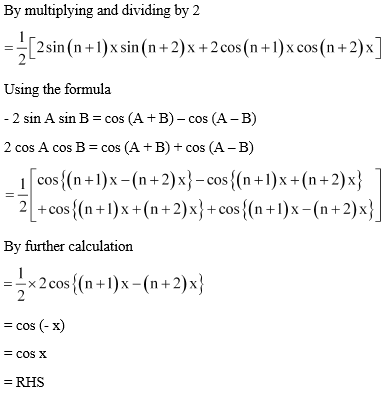Todays Deals### Chapter 3 Trigonometric Functions Ex-3.3 Contributorskrishan

Name:
Email:

# Latest News# 9000 interview questions in different categories